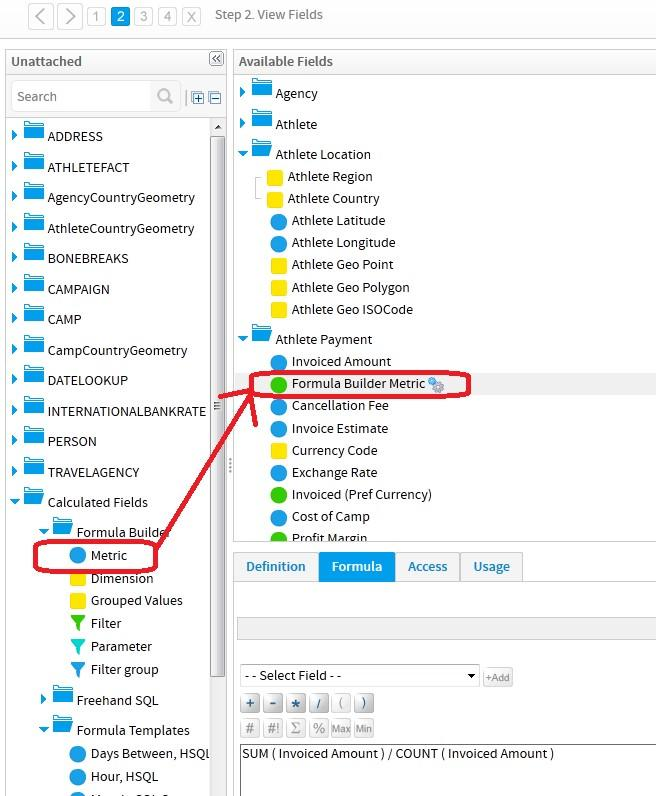# Forum

This content is now out of date.

# How to use different aggregate function in RATIO calculated field.

We want to add a computed measure in YF view which should be "Average Returns Per Transaction". This should ideally take into account SUM(NET_RETURNS) in the numerator and COUNT(RETURN_NUMBER) in the denominator.

We tried using calculated field "RATIO" but apparently within YF, SUM() divided by COUNT() will not work as they are two different aggregate functions. This however works very well in a SQL environment. As a workaround, we created a TEMP table to end up having both numerator & denominator as SUM(). This worked but is an overload on our processing for TEMP table creation & associated data population.

Is there a restriction as such within YF where we can't use separate aggregated functions together? If yes, is there any easy way out as an alternative or any other built-in function that we can use within YF?

Thanks,
Kishore
Hi Kishore,

it seems to me you were trying to use the Formula Template Calculated Field called Ratio (in the View Builder), and yes you are correct, the drop-down list of fields it shows does not include aggregations.

There are actually a few different ways you could create your calculated field of SUM(NET_RETURNS) / COUNT(RETURN_NUMBER). A quick summary of some of the possible choices would be a report-level calculated field, a view-level calculated field, a Custom Function, a Freehand SQL calculated field, and more...

However, seeing as it looks like you were wanting the calculation at the view-level then I'll show you how to do it using the view-level calculated field:

Go to Calculated Fields->Formula Builder->Metric and drag the Metric across into Available Fields then double-click it to open the definition then go to the Formula tab and choose the aggregations and fields you want:(N.B. the above example was done quickly, you may want to add a CASE statement to take into consideration the possibility of having zero in the denominator)

and here is that view-level calculated field used in a report:I hope that helps your reporting requirement, if there are further questions then please let us know.

regards,
Dave
Thanks David. It helped and report is working fine. Rather than using a straight forward Metric at View level to get the desired results, we were complicating the calculation by using calculated field "RATIO" from Formula Templates which fails when aggregated fields are used.

Thanks,
Kishore